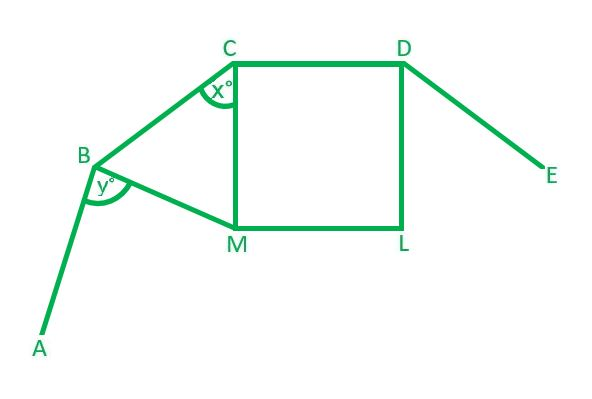# Finding Angle Measures

Geometry Level 3Given that $ABCDE$ in the above diagram is part of a regular polygon which has an interior angle of $160^\circ$, and $CDLM$ is a square, find the value of $x$ + $y$.

×

Problem Loading...

Note Loading...

Set Loading...